# Dividend Growth Rate

The percentage growth rate of a company’s dividend achieved during a certain period of time

## What is the Dividend Growth Rate?

The dividend growth rate (DGR) is the percentage growth rate of a company’s dividend achieved during a certain period of time. Frequently, the DGR is calculated on an annual basis. However, if necessary, it can also be calculated on a quarterly or monthly basis.The dividend growth rate is an important metric, particularly in determining a company’s long-term profitability. Since dividends are distributed from the company’s earnings, one can assess and analyze its ability to sustain its profitability by comparing the DGR over time.

### Dividend Growth Rate and a Security’s Pricing

Also, the dividend growth rate can be used in a security’s pricing. It is an essential variable in the Dividend Discount Model (DDM).

The dividend discount model is based on the idea that the company’s current stock price is equal to the net present value of the company’s future dividends. Mathematically, the dividend discount model is written using the following equation: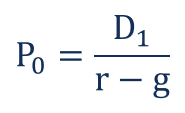Where:

• P0 – the current company’s stock price
• D1 – the next year dividends
• r – the company’s cost of equity
• g – the dividend growth rate

### How to Calculate the Dividend Growth Rate

The simplest way to calculate the DGR is to find the growth rates for the distributed dividends.

Let’s say that ABC Corp. paid its shareholders dividends of \$1.20 in year one and \$1.70 in year two. To determine the dividend’s growth rate from year one to year two, we will use the following formula: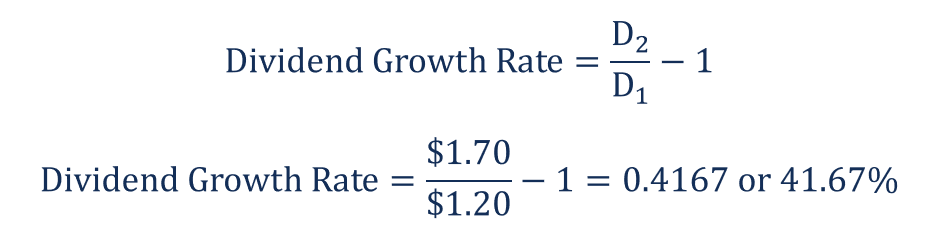However, in some cases, such as in determining the dividend growth rate in the dividend discount model, we need to come up with the forward-looking growth rate.

Prior to studying the approaches, let’s consider the following example. Below is ABC Corp.’s schedule of paid dividends with the calculated annual DGR: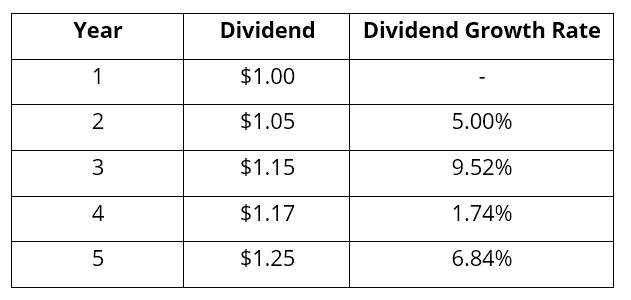There are three main approaches to calculate the forward-looking growth rate:

1. Use the historical dividend growth rates.

a. Using the historical DGR, we can calculate the arithmetic average of the rates: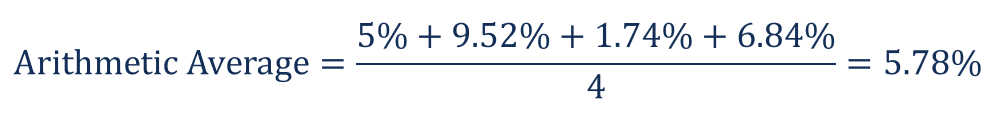b. We can also use the company’s historical DGR to calculate the compound annual growth rate (CAGR):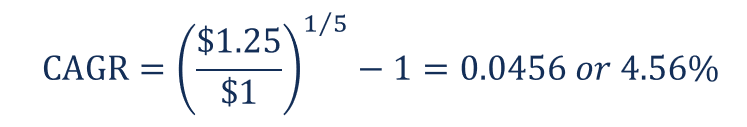2. Observe the dividend growth rate prevalent in the industry in which the company operates.

Imagine that the average DGR in the industry in which the ABC Corp. is operating is 4%. Then, we can use the said rate for ABC Corp.

1. Calculate the sustainable growth rate.

The sustainable growth rate is the maximum growth rate that a company can sustain without external financing. The sustainable growth rate can be found using the following formula: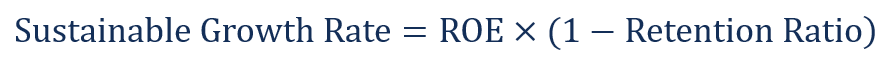If ABC Corp.’s ROE is 15% and its retention ratio is 65%, then the company’s sustainable growth rate will be: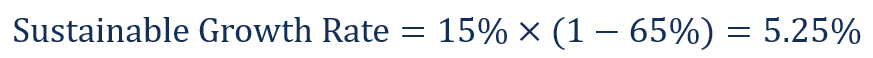### More Resources

CFI offers the Financial Modeling & Valuation Analyst (FMVA)™ certification program for those looking to take their careers to the next level. To keep learning and advancing your career, the following resources will be helpful:

• CAGR Calculator
• Capital Gains Yield
• Ex-Dividend Date
• Return on Total Capital

### Financial Analyst Training

Get world-class financial training with CFI’s online certified financial analyst training program!

Gain the confidence you need to move up the ladder in a high powered corporate finance career path.

Learn financial modeling and valuation in Excel the easy way, with step-by-step training.